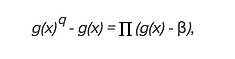### Algorithms

Polynomial FactorizationThe "Berlekamp algorithm" known to teachers of introductory algebra courses provides a quick and elegant way to factor polynomials over a small finite field of order q. The monic polynomial to be factored is f(x), of degree n. Its coefficients are known. The key idea is to find and exploit solutions, g(x), of the congruenceHere Q and I are n by n q-ary matrices. The entries of Q can be readily computed from the

polynomial f(x).

One then finds solution vectors, g, and corresponding polynomials, g. It is well-known thatBecause q is the order of the finite field, it is not hard to show that the coefficients of g satisfy a system of n linear equations,

(Q - I) g = 0.

where β runs over all q elements in the field. Since we now have a factorization of a multiple of f(x), we can factor f(x) by computing its gcd with each factor of the multiple.

This algorithm also provides a strong attack on factoring polynomials over the integers. Begin by factoring over Z, for some quite large integer n. Berlekamp  factored modulo a large prime; Zassenhaus factored modulo pᵐ, where p is a small prime and m is moderately large. Both methods are very fast for most polynomials, but there are rare polynomials which have many factors of small degrees modulo every prime-power, yet have few if any proper factors over Z. Lenstra later devised a method that handles these bad cases nicely.

Algebraic Coding Theory, McGraw-Hill, 1968; revised edition, Aegean Park Press, Laguna Hills, CA, 1984. See Chapter 6.

"Factoring Polynomials over Large Finite Fields", Mathematics of Computation 24:713-735 (1972); Math. Rev. 48:1943.

Algebraic DecodingThe "Berlekamp algorithm" best known to coding theorists is a fast way to invert matrices with constant diagonals. It works over any field, with the finite fields that occur in coding theory being the most popular. Massey's variation on the algorithm, used to synthesize linear feedback shift registers having a specified output sequence, became known as the "Berlekamp-Massey algorithm". The algorithm is also helpful for decoding various classes of algebraic codes. Since the goal is an algorithm whose work and storage requirements will be comparable to or better than ², the crucial step is to reformulate the problem in a way that avoids thinking about n by n matrices explicitly. This is done via this congruence:Here S(z) is the generating function for the terms along the successive diagonals of the traditional matrix. Its coefficients are the known inputs to the problem. σ(z) and ω(z) are the two polynomials (each of degree at most n) that will become the algorithm's outputs. The challenge was to find a faster algorithm to find the coefficients of these polynomials. The crux is an iterative procedure which successively computes polynomials that satisfy the "key equation". See Chapters 7 and 10 of Algebraic Coding Theory, McGraw-Hill, 1968; 3rd edition, World Scientific Press, 2014.

Implementations of that algorithm varied greatly in efficiencies. The special purpose hardware implemented in Cyclotomics' GF1 computer was by far the best.

For some applications with very high reliability requirements, most of the decoding effort was typically spent calculating the syndromes, S(z). Another Reed-Solomon decoding algorithm patented by Berlekamp and Welch in 1986 bypassed this problem, using parity checks much more easily recomputed by Berlekamp's 1983 Bit-Serial Encoder in lieu of the syndromes. Yet more refinements and improvements were presented in Berlekamp's 1996 paper, "Soft Decision+1 Reed-Solomon Decoding".

Some of these algorithms were applied to probabilistic checking of proofs by Madhu Sudan and his colleagues, who also devised ingenious algorithms to implement list decoding in a way which discovers all codewords that lie within some multiple of half the minimum distance. Although significantly slower than Welch-Berlekamp, Sudan's enhanced methods attain significantly improved performance when the code rate is low and the noise level is very high.

Bit-Serial EncodingThe figure depicts a portion of Berlekamp's Bit-Serial Reed-Solomon Encoder, which is NASA's standard for deep-space probes. Since the more complicated decoder is located on the ground, minimization of encoder power and weight is crucial. RS Encoders must perform many finite field multiplications, which in the 1970s were routinely implemented in a standard way. Berlekamp simplified the design enormously, yielding RS Encoders that are even simpler than encoders for cyclic binary codes of the same redundancy. One crucial step is the selection of a nonconventional basis for the representation of the field of order 2ᵐ over the field of order 2, a fact which almost contradicts the elementary theorem that the Galois field of order 2ᵐ is unique, and that all bases define the same field.

"Bit-Serial Reed-Solomon Encoders", IEEE Trans Inform Theory, IT28:869-874 (1982).